Brian Gervase

•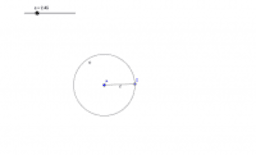Test

Activity

Brian Gervase

•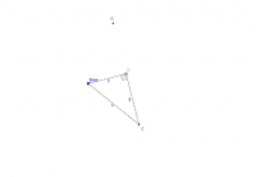right triangle

Activity

Brian Gervase

•Triangle Construction

Activity

Brian Gervase

•Construct Parallel Line

Activity

Brian Gervase

•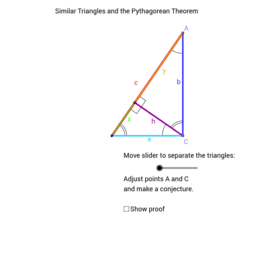Copy of Pythagorean and similar triangles.

Activity

Brian Gervase

•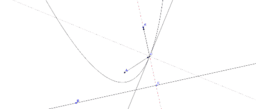parabola reflective

Activity

Brian Gervase

•Geometry Play

Activity

Brian Gervase

•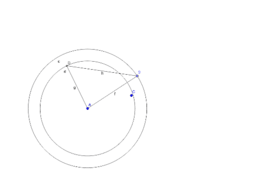Br

Activity

Brian Gervase

•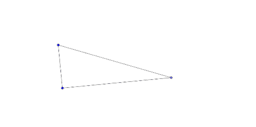Activity

Brian Gervase

•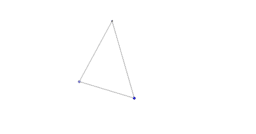What can you do with this triangle?

Activity

Brian Gervase

•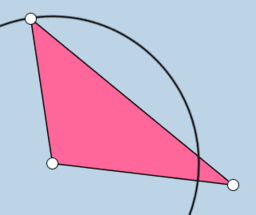Indentical Triangle: Construction

Activity

Brian Gervase

•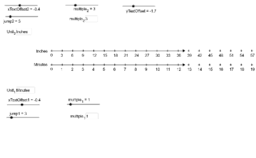Double Number Line Creation

Activity

Brian Gervase

•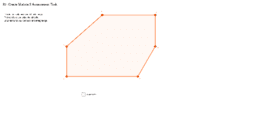Activity

Brian Gervase

•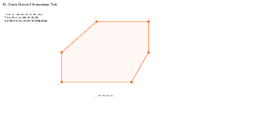Activity

Brian Gervase

•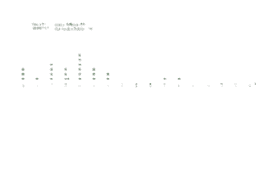Dot Plot Manipulative

Activity

Brian Gervase

•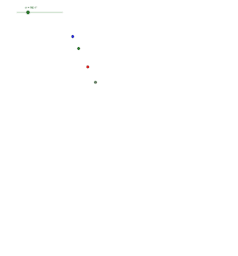Are they all moving the same speed?

Activity

Brian Gervase

•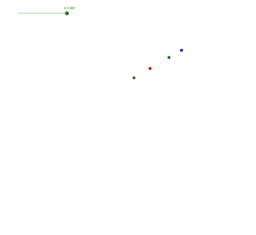Activity

Brian Gervase

•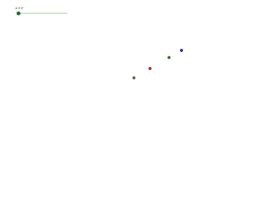Are they moving the same speed?

Activity

Brian Gervase

•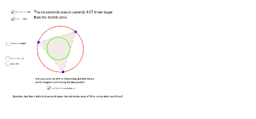Two Triangle Circles

Activity

Brian Gervase

•Two Triangle Circles

Activity

Brian Gervase

•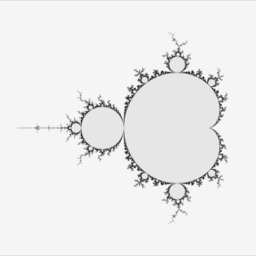GeoGebra Workshop Exemplar Book

Book

Brian Gervase

•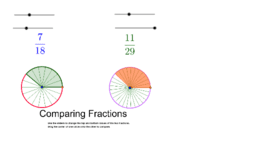Fraction Wheels

Activity

Brian Gervase

•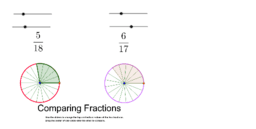Fraction Wheels

Activity

Brian Gervase

•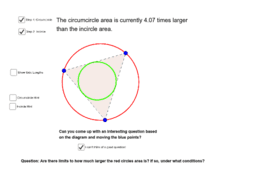Two Triangle Circles

Activity

Brian Gervase